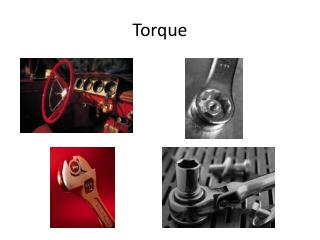DownloadDownload PresentationTorque

# Torque

Télécharger la présentation## Torque

- - - - - - - - - - - - - - - - - - - - - - - - - - - E N D - - - - - - - - - - - - - - - - - - - - - - - - - - -
##### Presentation Transcript

1. Torque

2. Torque ≡ the action of a leveraged force • Torque is the ability of a force to cause rotation or a turning motion. • Unbalanced torque provides a rotational acceleration. • Torque τ has two components: a perpendicularly applied force, F, and a leverage arm distance, r • Torque equation: τ = F∙r • SI unit of measure for torque is: N∙m

3. Torque sample calculation #1 • How much torque would you produce by applying 20 N of force perpendicular to a 25 cm long wrench? F = 20N and r = .25m τ = ? • Answer: τ = (20 N)(.25 m) = 5.0 N∙m

4. Torque sample calculation #2 • How much is the minimum force needed to be applied to a .10 m long door handle if the handle requires 12 N∙m of torque to be turned? r = .10 m and τ = 12 N∙m F = ? • Answer: F = τ/r = 12 N∙m/.10 m = 120 N

5. Torque sample question #3 • Q: By what factor does the torque change if you double the leverage arm while keeping the force the same? A: Double

6. Ways to change the amount of torque • There are two ways to change the amount of torque: 1) change the amount of force applied or 2) change the leverage arm length. • Various tools (ex: wrenches) and devices (ex: wheels) often use a longer leverage length to reduce the amount of applied force needed.

7. Balanced torque • A seesaw is an example of an object that can easily demonstrate balanced torque or rotationalequilibrium. The torque on the left = torque on the right = 100 N∙m